# Complex numbers + absolute value - math problems

#### Number of problems found: 7

• Distance two imaginary numbs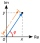Find the distance between two complex number: z1=(-8+i) and z2=(-1+i).
• Modulus and argumentFind the mod z and argument z if z=i
• The modulus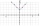Find the modulus of the complex number 2 + 5i
• Moivre 2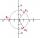Find the cube roots of 125(cos 288° + i sin 288°).
• Log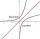Calculate value of expression log |3 +7i +5i2| .
• Goniometric form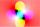Determine the goniometric form of a complex number z = √ 110 +4 i.
• ABS CNCalculate the absolute value of complex number -15-29i.

We apologize, but in this category are not a lot of examples.
Do you have an exciting math question or word problem that you can't solve? Ask a question or post a math problem, and we can try to solve it.

We will send a solution to your e-mail address. Solved examples are also published here. Please enter the e-mail correctly and check whether you don't have a full mailbox.

Try our complex numbers calculator. Complex numbers - math problems. Absolute value - math problems.